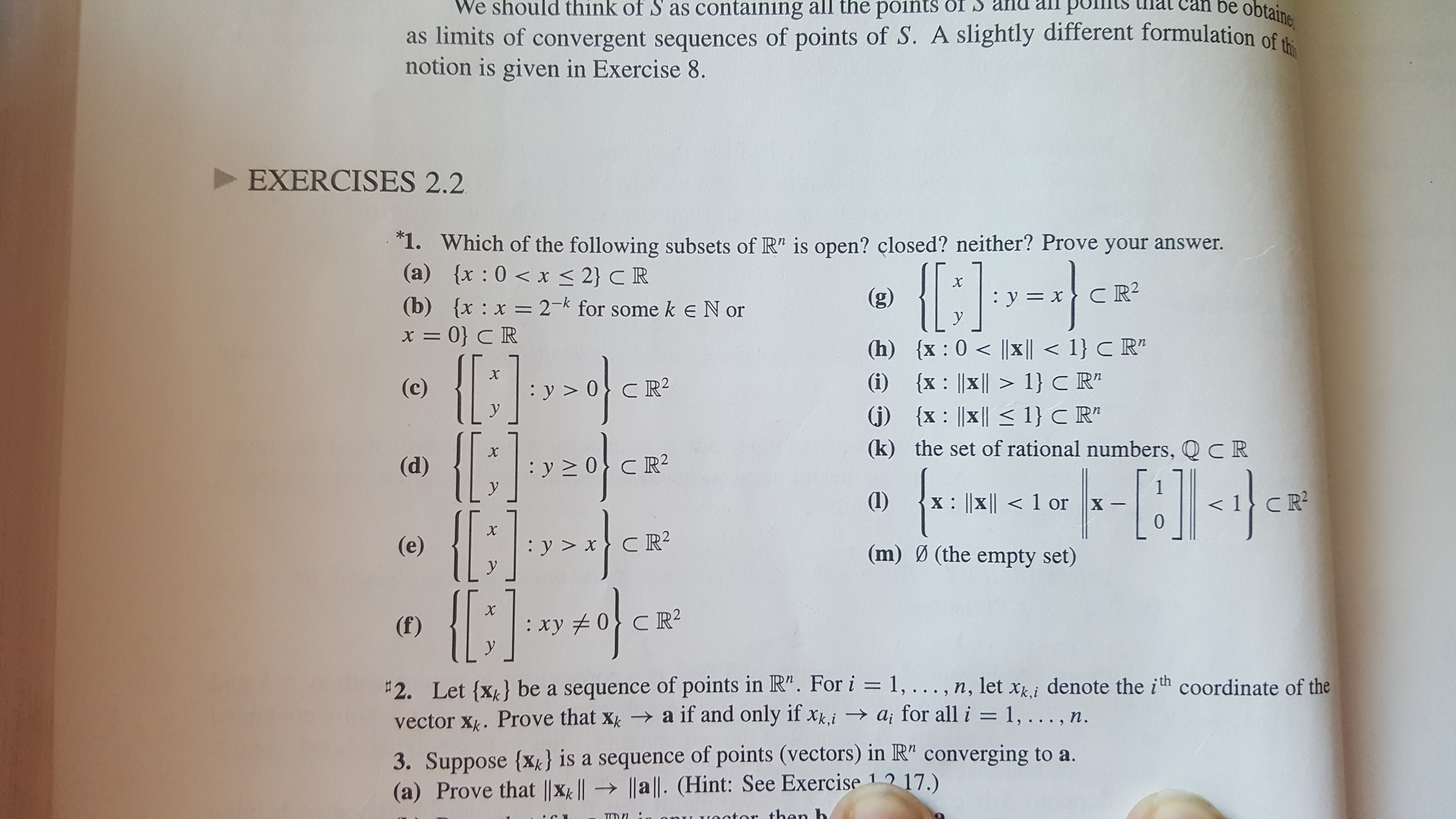# We should think of S as containing all the points OI S anu all putsas limits of convergent sequences of points of S. A slightly different formulation of thnotion is given in Exercise 8.an be obtaineEXERCISES 2.21. Which of the following subsets of R" is open? closed? neither? Prove your answer.(a) x : 0x < 2} C R(b) {x x 2- for some keN orx= 0} C R(g):y=xCR: X =y(h) x: 0 x |1}C R"(i) x x 1} C R"j x |x 1} C R"(k) the set of rational numbers, Q CRX(c)C R2yX(d)y 2уCR21(1)X: ||X||хУC R2(m) (the empty set)X(f): ху 2УCR22. Let {x} be a sequence of points in R". For i = 1, .. . , n, 1let xi denote the ith coordinate of thevector Xk. Prove that xk a if and only if xk,iai for all i = 1, .. . , n.3. Suppose {x} is a sequence of points (vectors) in R" converging to a.(a) Prove that |Xk |l a . (Hint: See Exercise 1 17.)

Question
3 viewshelp_outlineImage TranscriptioncloseWe should think of S as containing all the points OI S anu all puts as limits of convergent sequences of points of S. A slightly different formulation of th notion is given in Exercise 8. an be obtaine EXERCISES 2.2 1. Which of the following subsets of R" is open? closed? neither? Prove your answer. (a) x : 0x < 2} C R (b) {x x 2- for some keN or x= 0} C R (g) : y=xCR : X = y (h) x: 0 x |1}C R" (i) x x 1} C R" j x |x 1} C R" (k) the set of rational numbers, Q CR X (c) C R2 y X (d) y 2 у CR2 1 (1) X: ||X||<1 or X CR2 X (e) : у>х У C R2 (m) (the empty set) X (f) : ху 2 У CR2 2. Let {x} be a sequence of points in R". For i = 1, .. . , n, 1let xi denote the ith coordinate of the vector Xk. Prove that xk a if and only if xk,iai for all i = 1, .. . , n. 3. Suppose {x} is a sequence of points (vectors) in R" converging to a. (a) Prove that |Xk |l a . (Hint: See Exercise 1 17.) fullscreen
check_circle

Step 1

Given:

...

### Want to see the full answer?

See Solution

#### Want to see this answer and more?

Solutions are written by subject experts who are available 24/7. Questions are typically answered within 1 hour.*

See Solution
*Response times may vary by subject and question.
Tagged in

### Math